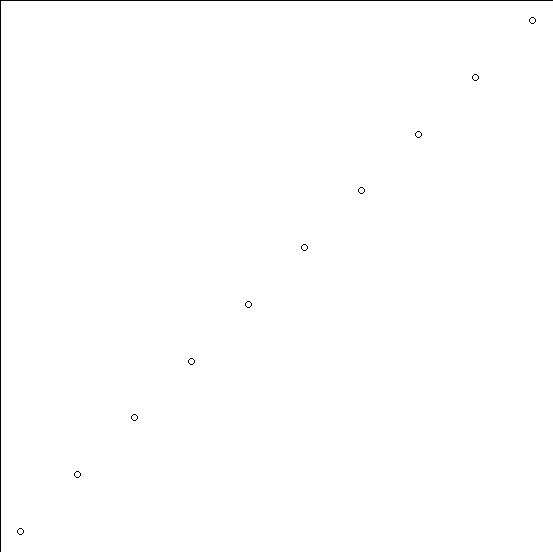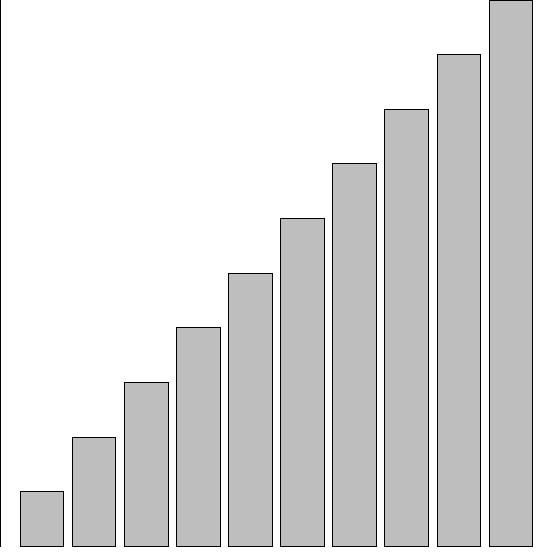# How to create a plot in base R without margins?

To create a plot without margins, we first need to define that margin in a way that the plot created after that will not have margins and this can be done by using par function. We would need to pass mar function within par function as par(mar=c(0,0,0,0)).

## Example

Live Demo

par(mar=c(0,0,0,0))
plot(1:10)

## Output## Example

Live Demo

barplot(1:10)

## OutputUpdated on: 07-Dec-2020

294 Views